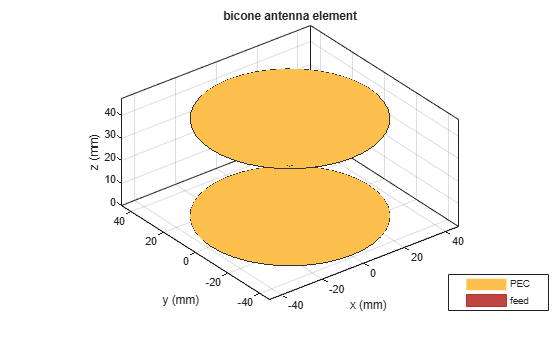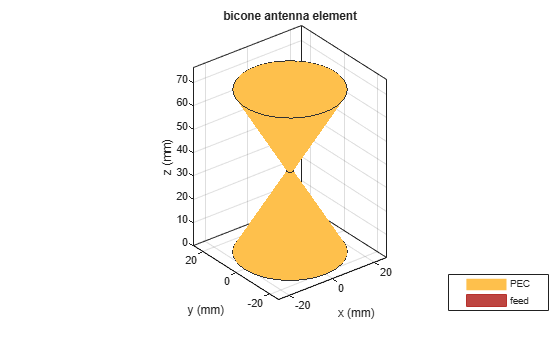# coneangle2size

## Syntax

``conedimensions = coneangle2size(slantheight,halfconeangle,Name,Value)``

## Description

example

````conedimensions = coneangle2size(slantheight,halfconeangle,Name,Value)` calculates the equivalent cone height,broad radius, and narrow radius for a cone from its half cone angle,slant length, and either feedwidth or narrow radius```

## Examples

collapse all

Calculate the cone height, the broad radius, and the narrow radius of the cone in a bicone antenna using a half cone angle of 30 degrees, slant length of 0.0400 m, feed width of 0.001 m.

```ant = bicone('FeedHeight',0.3e-3,'FeedWidth',0.5e-3); show(ant)````m = coneangle2size(30,40e-3,'FeedWidth',1e-3)`
```m = struct with fields: NarrowRadius: 0.0013 BroadRadius: 0.0213 ConeHeight: 0.0346 ```
```ant.ConeHeight = m.ConeHeight; ant.BroadRadius = m.BroadRadius; ant.NarrowRadius = m.NarrowRadius; show(ant)```## Input Arguments

collapse all

Length from the base of the cone to point on the circle with the narrow radius, specified as a positive scalar in meters.

Data Types: `double`

Half of the cone angle, specified as a positive scalar in degrees. This value must be between 5 and 85 degrees.

Data Types: `double`

### Name-Value Arguments

Specify optional pairs of arguments as `Name1=Value1,...,NameN=ValueN`, where `Name` is the argument name and `Value` is the corresponding value. Name-value arguments must appear after other arguments, but the order of the pairs does not matter.

Before R2021a, use commas to separate each name and value, and enclose `Name` in quotes.

Example: `'FeedWidth' = 0.02`

Width of the feed, specified as the comma-separated pair consisting of `'FeedWidth'` and a positive scalar in meters.

Data Types: `double`

Radius at the apex of the cone, specified as the comma-separated pair consisting of `'NarrowRadius'` and a positive scalar in meters.

Data Types: `double`

## Version History

Introduced in R2020a